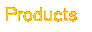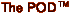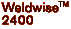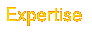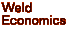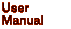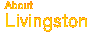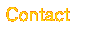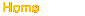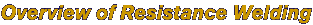Historical Background Introduction Variables in the Welding Process Critical Factors in Welding ----------------- Electrodes, Surface Contact and Current Density Ohm's and Joule's Laws Identifying and Correcting Weld Problems Industry Resource Addresses Right click here to download a complete PDF of the Rev.C Livingston POD User's Manual.
 Critical Factors in Welding Understanding the resistance weld process requires an understanding of the main factors involved and how they work together. This section will review current, voltage, resistance, and power, as well as the various functions of the electrodes and how they affect surface contact and current density. Current Current, usually measured in Kilo-Amperes (KA - one Kilo-Amp is equal to 1,000 Amps), is one of the most important factors. A resistance weld cannot be made unless there is sufficient weld current. According to the RWMA, the typical amount of current needed to weld low-carbon steel, for example, is about 10,000 Amps (10 kA) at about 5 Volts. To put this in perspective, a normal household or office outlet provides a maximum of 15-20 Amps (0.015-0.020 kA) at 120 Volts, while a power circuit in a factory may only be capable of providing 200 Amps (0.200 kA) at 500 Volts to a welder. The factory's 200 Amps is then converted to the 10,000 Amps needed to weld by means of a welding transformer. A transformer consists of two coils of wire, called the primary and the secondary, wound around an iron core. Power is transferred from primary to secondary via the magnetic properties of the iron. The factor by which the current and voltage is stepped up or down is equal to the ratio between the number of turns of wire in the coils forming the primary and secondary windings of the transformer. Consider the steel that needs 10,000 Amps (10 kA) of current to be welded in a factory that can only provide 200 Amps (0.200 kA). If the welding transformer had 100 turns on the primary and 2 turns on the secondary, the 'turns ratio' would be 100 to 2, or more simply, 50 to 1. The 200 Amp current in the primary would then be converted (stepped up) to 10,000 Amps (200 Amps x 50 turns = 10,000 Amps) in the secondary, which would yield enough amperage to make a weld. Voltage If current is the amount of electricity flowing, then Voltage (measured in Volts) is the pressure or force that's causing the flow. A good analogy is water flowing through a pipe. A larger voltage will result in greater water pressure, which will cause more water (current) to flow through the pipe. Using the transformer example above, after the 200 Amps at 500 Volts on the primary passes through the transformer coils, the secondary amperage increases to 10,000 Amps, but the voltage actually drops to 10 Volts. This decrease in voltage occurs because the amount of power coming out of a transformer isn't actually increased, but more accurately exchanged. Power Power is Voltage multiplied by Current, and is measured in Watts, or KVA (KVA stands for Kilo-Volt-Amperes. Watts and KVA will be used interchangeably in this text). This means that the amount of current flowing times the pressure that's causing it to flow equals the amount of power generated. A basic law to bear in mind is that the power going into a transformer will always equal the power coming out of it. Returning to the transformer example, 200 Amps coming in at 500 Volts (200 x 500 = 100,000 KVA) on the primary with a 50 to 1 turns ratio in the transformer will be converted into 10,000 Amps at 10 Volts (10,000 x 10 = 100,000 KVA) going out. As the math illustrates, the results are the same. The initial and final amperage and voltage may be different, but because the ratio is the same, the total amount of power is also the same. Resistance As mentioned earlier, resistance is defined as the opposition that a substance offers to the flow of electric current. Resistance is calculated by dividing the Voltage by the Current, and is measured in Ohms. (When written, Ohms are represented by the Greek letter Omega: .). Since resistance to the current is what generates the heat in the workpiece, it is critically important that the area with the greatest resistance be at the interface between the two parts being joined. This interface is also known as the faying surfaces. Remember that the heat is where the resistance is, and the resistance is where the heat will be. If the area with the most resistance is, for example, where the lower bus bar connects to the transformer of the welder and not at the faying surfaces of the workpieces, then that's where the heat will go. Likewise, if the greatest resistance is at the contact area between the electrode tip and the workpiece, the heat generated there will cause the tip to weld directly to the workpiece.